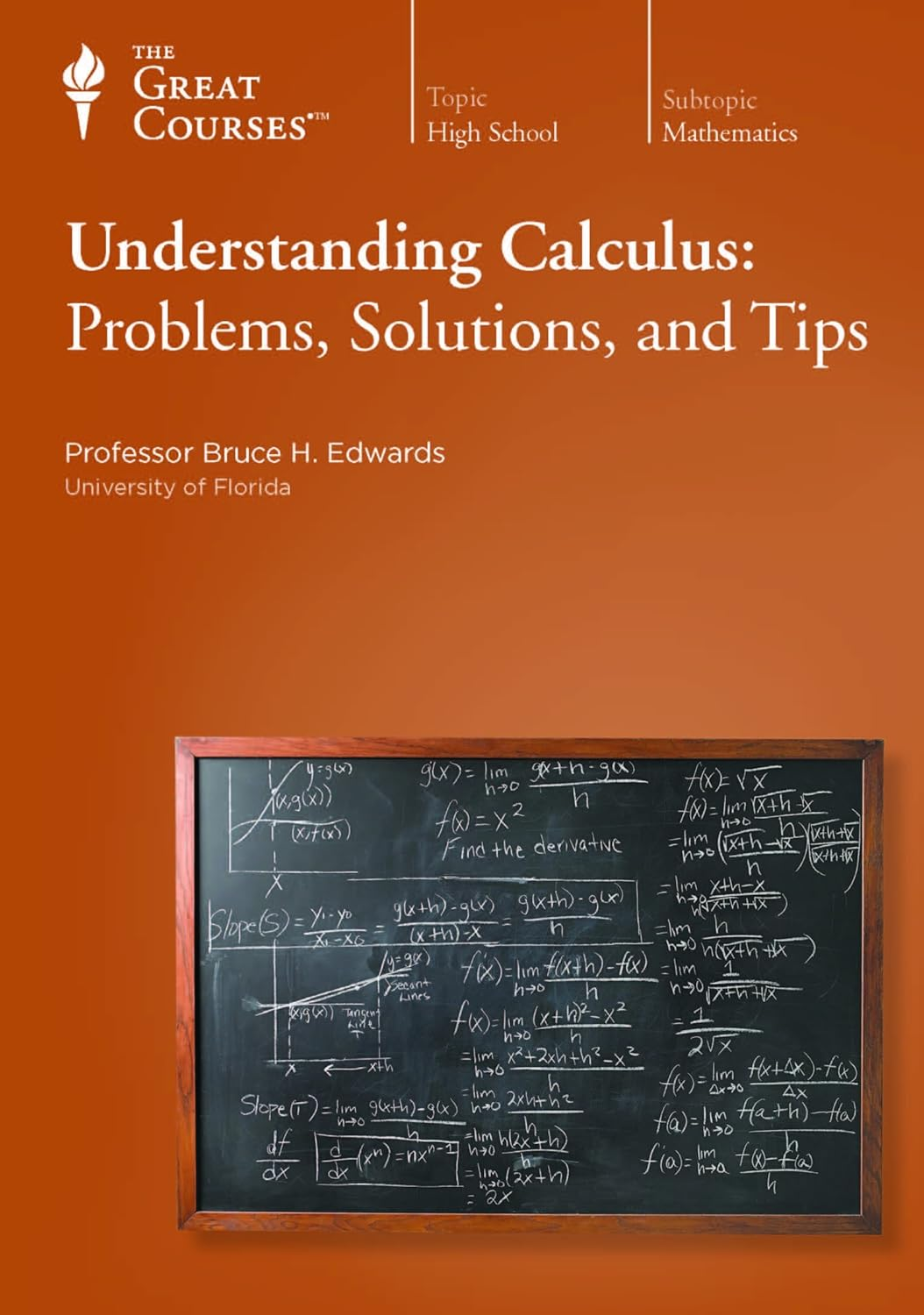TTC - Understanding Calculus: Problems, Solutions, and Tips [repost]immerses you in the unrivaled learning adventure of this mathematical field in 36 half-hour lectures that cover all the major topics of a full-year calculus course in high school at the College Board Advanced Placement AB level or a first-semester course in college. With crystal-clear explanations of the beautiful ideas of calculus, frequent study tips, pitfalls to avoid, and-best of all-hundreds of examples and practice problems that are specifically designed to explain and reinforce major concepts, this course will be your sure and steady guide to conquering calculus.

Your teacher for this intensively illustrated DVD set is Professor Bruce H. Edwards, an award-winning instructor at the University of Florida and the coauthor of a best-selling series of calculus textbooks.

Accomplish Mathematical Wonders
Calculus is one of the most powerful and astonishing tools ever invented, yet it is a skill that can be learned by anyone with an understanding of high school mathematics.

Among its many uses, calculus teaches you to

analyze a multitude of situations involving change, whether it's an accelerating rocket, the growth of a bacterial colony, or fluctuating stock prices;
calculate optimum values, such as the greatest volume for a box with a given surface area or the highest feasible profit from the sales of an item;
measure complex shapes-for example, the volume of a doughnut-shaped object called a torus or the area of a plot of land bounded by a river.

Learn about Precalculus and Limits . . .
Solving many types of calculus problems usually requires employing precalculus-algebra and trigonometry-to work out a solution. For this reason, Professor Edwards devotes the first few lectures to reviewing key topics in precalculus, then he covers some basic concepts such as limits and continuity before moving on to the two simple, yet brilliant ideas behind calculus-the derivative and the integral.

Despite the apparent differences between the derivative and integral, you discover that they are inextricably linked by the surprising fundamental theorem of calculus. Throughout the course, you will discover that simplicity is one of the hallmarks of the essential ideas of calculus.

. . . the Power of the Derivative . . .
The derivative is the foundation of differential calculus, which you study through Lecture 17, exploring its many applications in science, engineering, business, and other fields.

You start with a classic problem that illustrates one of the core ideas of calculus: Can you find the tangent line to a curve at a given point? This is the same as asking if the rate of change of the curve can be measured at that point-with a host of potential applications in situations where a quantity is changing, such as the speed of an accelerating vehicle. The answer is: Yes, and with amazing simplicity! After learning the steps involved, you have solved your first calculus problem.

You then

study a variety of ways to find derivatives, including the power rule, the constant multiple rule, the quotient rule, the chain rule, and implicit differentiation;
learn how to find extrema-the absolute maximum and minimum values of functions, using derivatives; and
apply derivatives to solve a variety of real-world problems.
. . . and the Importance of the Integral
Next, you are introduced to the integral, using a classic problem in which you are asked to find the area of a plot of land bounded by curves. To solve this problem, calculus provides us with the integral-a powerful tool that allows us to calculate areas, volumes, and other characteristics of complex shapes. The balance of the course is devoted to integral calculus and its applications. You study

arc length and surface area-two applications of calculus that are at the heart of engineering;
integration by substitution-a method that enables you to convert a difficult problem into one that's easier to solve; and
the formulas for continuous compound interest, radioactive decay, and a host of other real-world applications.

A Calculus Course for All

Understanding Calculus is well suited for anyone who wants to take the leap into one of history's greatest intellectual achievements, whether for the first time or for review. Those who will benefit include these learners:

Any student now studying calculus who would like personal coaching from a professor who has spent years honing his explanations for the areas that are most challenging to students. This course is specifically designed to cover all the major topics of a full-year calculus course in high school at the College Board Advanced Placement AB level or a first-semester course in college.
Parents of students studying calculus, a subject with which they often give up trying to help their high-school-age children-at a critical turning point in their educational careers.

[Fast Download] TTC - Understanding Calculus: Problems, Solutions, and Tips [repost]